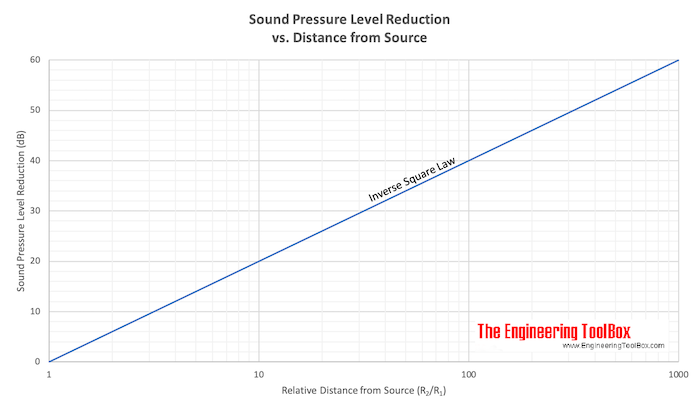Engineering ToolBox - Resources, Tools and Basic Information for Engineering and Design of Technical Applications!

# Sound Propagation - the Inverse Square Law

## Doubling of the distance from a noise source reduces the sound pressure level with 6 decibel.

In a free field - a doubling of the distance from a noise source reduces the sound pressure level with 6 decibel.

This - the Inverse Square Law - can be expressed in a diagram likeDownload and print the Inverse Square Law chart - Sound pressure level reduction vs. distance from source

dL = Lp2 - Lp1

= 10 log (R2 / R1)2

=  20 log (R2 / R1)                                        (1)

where

dL = difference in sound pressure level (dB)

Lp1 = sound pressure level at location 1 (dB)

Lp2 = sound pressure level at location 2 (dB)

R1 = distance from source to location 1 (ft, m)

R2 = distance from source to location 2 (ft, m)

A "free field" is defined as a flat surface without obstructions.

#### Example - Rifle Shot and Sound Pressure at Distance

If the sound pressure from a rifle shot is measured to 134 dB at 1.25 feet - the reduction in sound pressure level at distance 80 feet can be calculated as

dL = 20 log ((80 ft) / (1.25 ft))

= 36 dB

The sound pressure level at distance 80 ft can be calculated as

Lp2 = (134 dB) - (36 dB)

= 98 dB

Distance
(feet)
Sound Pressure
Lp
(decibel)
1.25 134
2.5 128
5 122
10 116
20 110
40 104
80 98
160 92
320 86
640 78
1280 74
2560 68
5120 62

### Inverse Square Law Calculator

Use the calculator below to calculate the sound pressure level at distance.

Lp1 - sound pressure level at location 1 (dB)

R1 - distance from source to location 1 (m, ft)

R2 - distance from source to location 2 (m, ft)

### Example - Noise from a Machine

The noise from a machine in distance 1 m is measured to 110 dB. The noise reduction due to the inverse square law to a working area at distance 5 m can be calculated as

dL = 20 log ((5 m) / (1 m))

= 14 dB

The sound pressure level in the working area can be calculated as

Lp2 = (110 dB) - (14 dB)

= 96 dB

This noise level is only permitted for a limited amount of time and some action with partial barriers or enclosure of the machine should be done.

## Related Topics

• Acoustics - Room acoustics and acoustic properties, decibel A, B and C, Noise Rating (NR) curves, sound transmission, sound pressure, sound intensity and sound attenuation.

## Engineering ToolBox - SketchUp Extension - Online 3D modeling!

Add standard and customized parametric components - like flange beams, lumbers, piping, stairs and more - to your Sketchup model with the Engineering ToolBox - SketchUp Extension - enabled for use with the amazing, fun and free SketchUp Make and SketchUp Pro .Add the Engineering ToolBox extension to your SketchUp from the SketchUp Pro Sketchup Extension Warehouse!

Translate

## Privacy

We don't collect information from our users. Only emails and answers are saved in our archive. Cookies are only used in the browser to improve user experience.

Some of our calculators and applications let you save application data to your local computer. These applications will - due to browser restrictions - send data between your browser and our server. We don't save this data.

## Citation

• Engineering ToolBox, (2005). Sound Propagation - the Inverse Square Law. [online] Available at: https://www.engineeringtoolbox.com/inverse-square-law-d_890.html [Accessed Day Mo. Year].

Modify access date.

. .

#### Scientific Online Calculator3 30